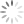Loading, please wait...## Memory Management

The exact size of the array is unknown until the compile time, i.e., a time when a compiler compiles code written in a programming language into an executable form. The size of the array you have declared initially can be sometimes insufficient and sometimes more than required. Dynamic memory allocation allows a program to obtain more memory space while running or to release space when no space is required.

Although, C language inherently does not has any technique to allocated memory dynamically, there are four library functions under "stdlib.h" for dynamic memory allocation.

Function

Use of Function

malloc()

Allocates requested size of bytes and returns a pointer first byte of allocated space

calloc()

Allocates space for array elements, initialize to zero and then returns a pointer to memory

free()

Deallocate the previously allocated space

realloc()

Change the size of previously allocated space

## malloc()

The name malloc stands for "memory allocation". The function malloc() reserves a block of memory of specified size and return a pointer of type void which can be cast into a pointer of any form.

### The syntax of malloc()

``ptr=(cast-type*)malloc(byte-size)``

Here,  ptr is a pointer of cast-type. The malloc() function returns a pointer to an area of memory with the size of byte size. If the space is insufficient, allocation fails and returns a NULL pointer.

Example

``Ptr=(int*)malloc(100*sizeof(int));``

This statement will allocate either 200 or 400 according to the size of int 2 or 4 bytes respectively and the pointer points to the address of the first byte of memory.

## calloc()

The name calloc stands for "contiguous allocation". The only difference between malloc() and calloc() is that malloc() allocates a single block of memory whereas calloc() allocates multiple blocks of memory each of the same size and sets all bytes to zero.

### Syntax of calloc()

``Ptr=(cast-type*)calloc(n,element-size);``

This statement will allocate contiguous space in memory for an array of n elements.

For example:

``Ptr=(float*)calloc(25,sizeof(float));``

This statement allocates contiguous space in memory for an array of 25 elements each of size of float, i.e, 4 bytes.

## free()

Dynamically allocated memory with either calloc() or malloc() does not get a return on its own. The programmer must use free() explicitly to release space.

### syntax of free()

``free(ptr);``

This statement cause the space in memory pointer by ptr to be deallocated.

## Examples of calloc() and malloc()

C program to find the sum of n elements entered by the user. To perform this program, allocate memory dynamically using malloc() function.

`````` /*How to use malloc() in a C program*/.
#include <stdio.h>
#include <stdlib.h>
int main(){
int n,i,*ptr,sum=0;
printf("Enter number of elements: ");
scanf("%d",&n);
ptr=(int*)malloc(n*sizeof(int)); //memory allocated using malloc if(ptr==NULL)
{
printf("Error! memory not allocated.");
exit(0);
}
printf("Enter elements of array: ");
for(i=0;i<n;++i)
{
scanf("%d",ptr+i);
sum+=*(ptr+i);
}
printf("Sum of the elements of Array=%d",sum);
free(ptr);
return 0;
}``````

## Example

``````/*C program for how to use calloc()*/

#include <stdio.h>
#include <stdlib.h>
int main()
{
int n,i,*ptr,sum=0;
printf("Enter number of elements: ");
scanf("%d",&n);
ptr=(int*)calloc(n,sizeof(int));
if(ptr==NULL)
{
printf("Error! memory not allocated.");
exit(0);
}
printf("Enter elements of array: ");
for(i=0;i<n;++i)
{
scanf("%d",ptr+i);
sum+=*(ptr+i);
}
printf("Sum=%d",sum);
free(ptr);
return 0;
}``````

## realloc()

If the previously allocated memory is insufficient or more than sufficient. Then, you can change memory size previously allocated using realloc().

### Syntax of realloc()

``ptr=realloc(ptr,newsize);``

Here, ptr is reallocated with the size of newsize.

``````/*A C program for how to use realloc()*/

#include <stdio.h>
#include <stdlib.h>
int main()
{
int *ptr,i,num1,num2;
printf("Enter size of array: ");
scanf("%d",&num1);

ptr=(int*)malloc(num1*sizeof(int));

printf("Address of previously allocated memory: ");

for(i=0;i<n1;++i) printf("%u\t",ptr+i);

printf("\nEnter new size of array: ");

scanf("%d",&num2);

ptr=realloc(ptr,num2);

for(i=0;i<num2;++i)

printf("%u\t",ptr+i);

return 0;
}``````